# Gram-Charlier series

(diff) ← Older revision | Latest revision (diff) | Newer revision → (diff)

A series defined by the expression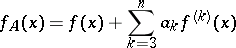(1)

or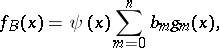(2)

whereis the normalized value of a random variable.

The series (1) is known as the Gram–Charlier series of type; here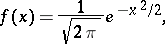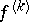is the-th derivative of, which can be represented aswhere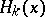are the Chebyshev–Hermite polynomials. The derivativesand the polynomialsare orthogonal, owing to which the coefficients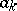can be defined by the basic moments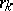of the given distribution series. If one restricts to the first few terms of the series (1), one obtains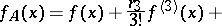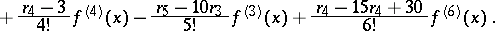The series (2) is known as a Gram–Charlier series of type; here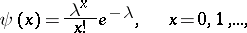while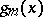are polynomials analogous to the polynomials.

If one restricts to the first terms of the series (2), one obtains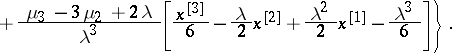Hereare the central moments of the distribution, while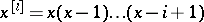.

Gram–Charlier series were obtained by J.P. Gram  and C.V.L. Charlier  in their study of functions of the formThese are convenient for the interpolation between the values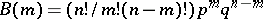of the general term of the binomial distribution, where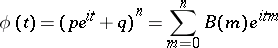is the characteristic function of the binomial distribution. The expansion of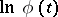in powers ofyields a Gram–Charlier series of typefor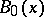, whereas the expansion ofin powers ofyields a Gram–Charlier series of type.

How to Cite This Entry:
Gram-Charlier series. Encyclopedia of Mathematics. URL: http://encyclopediaofmath.org/index.php?title=Gram-Charlier_series&oldid=18126
This article was adapted from an original article by A.K. Mitropol'skii (originator), which appeared in Encyclopedia of Mathematics - ISBN 1402006098. See original article